# Customizing superb plots

Creating a plot involves reading raw data and compiling these into summary statistics. This step is handled by superb transparently. The second, more involving step, however is to customize the plots so that it looks appealing to the readers.

In this vignette, we go rapidly over superb functionalities. Instead, we provide a worked-out example to produce a fully customized rain-drop plot. We proceed with examples taken from scientific articles.

In the following, we need the following libraries:

## Load relevant packages
library(superb)             # for superbPlot
library(ggplot2)            # for all the graphic directives
library(gridExtra)          # for grid.arrange

If they are not present on your computer, first upload them to your computer with install.packages("name of the package").

# Figure 2 of Hofer, Langmann, Burkart and Neubauer, 2022.

In their study, Hofer, Langmann, Burkart, & Neubauer (2022) examined who is the best judges of one’s abilities. Examining self-ratings vs.  other-ratings in six domain, they found out that we are not always the best judges. They present in their Figure 2 a rain-cloud plot (Allen, Poggiali, Whitaker, Marshall, & Kievit (2019)) illustrating the ratings.

In what follow, we discuss how this plot could be customized after its initial creation with superb.

As the six domains are within-subject ratings, the data must be composed of 6 columns (at least, there can be additional columns; they won’t be illustrated herein). In case you do not have such data, the following subsection generates mock data.

## Generating mock data

We generate two sets of mock data from six sets of means and standard deviations:

Astats <- data.frame(
MNs = c(6.75, 6.00, 5.50, 6.50, 8.00, 8.75),
SDs = c(2.00, 3.00, 3.50, 3.50, 1.25, 1.25)
)
dtaA <- apply(Astats, 1,
function(stat) {rnorm(100, mean=stat, sd=stat)}
)
dtaA <- data.frame(dtaA)
colnames(dtaA) <- c("Verbal", "Numerical", "Spatial", "Creativity", "Intrapersonal", "Interpersonal")

Bstats <- data.frame(
MNs = c(3.33, 3.00, 2.50, 3.00, 2.75, 3.50),
SDs = c(0.25, 0.50, 0.66, 0.50, 0.25, 0.25)
)
dtaB <- apply(Bstats, 1,
function(stat) {rnorm(100, mean=stat, sd=stat)}
)
dtaB <- data.frame(dtaB)
colnames(dtaB) <- c("Verbal", "Numerical", "Spatial", "Creativity", "Intrapersonal", "Interpersonal")

The datasets are data.frames called dtaA and dtaB. Their columns names are the dependent variables, e.g., “Verbal”, “Numerical”, “Spatial”, “Creativity”, “Intrapersonal”, “Interpersonal”.

## Making the top-row plot

For convenience, we make lists of the desired colors and labels we want to appear on the x-axis:

mycolors <- c("seagreen","chocolate2","mediumpurple3","deeppink","chartreuse4", "darkgoldenrod1")
mylabels <- c("Verbal", "Numerical", "Spatial", "Creativity", "Intrapersonal", "Interpersonal")

pltA <- superbPlot(dtaA,        # plot for the first data set...
WSFactors = "Domain(6)",    # ...a within-subject design with 6 levels
variables = mylabels,       # ...whose variables are contained in the above list
purpose = "difference", # we want to compare means
decorrelation = "CM"    # and error bars are correlated-adjusted
),
plotStyle="raincloud",

# the following (optional) arguments are adjusting some of the visuals
pointParams    = list(size = 0.75),
jitterParams   = list(width =0.1, shape=21,size=0.05,alpha=1), # less dispersed jitter dots,
violinParams   = list(trim=TRUE, alpha=1),                     # not transparent,
errorbarParams = list(width = 0.1, size=0.5)                   # wider bars, thicker lines.
)
## Warning in (function (mapping = NULL, data = NULL, stat = "identity", position =
## "identity", : Ignoring unknown parameters: size
pltA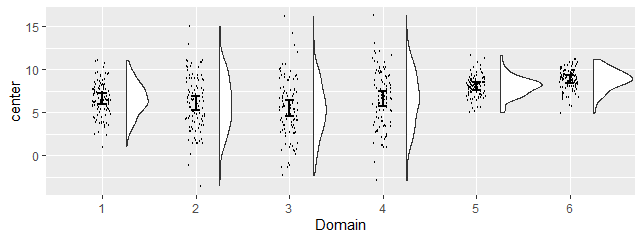Figure 2, preliminary version

As seen, this plot is a standard, colorless, plot. It contains all that is needed; it is just plain drab and the labels are generic ones (on the vertical axis and on the horizontal axis).

## Adding a color layer to the plot

Using superb, if there is only one factor, superb will consider that it is the one on the x-axis and there is therefore no other layers in the plot. This is why the current plot is colorless.

It is possible, post-hoc, to indicate that we wish additional layers in the plot.

In the present, we want to add the fill and the color of dots layers. These layers are to be “connected” to the sole factor in the present example (that is, Domain). Consequently, the x-axis labels, the fill color and the dot color are all redondant information identifying the condition.

To do this, simply add an aesthetic graphic directive to pltA with:

pltA + aes(fill = factor(Domain), colour = factor(Domain))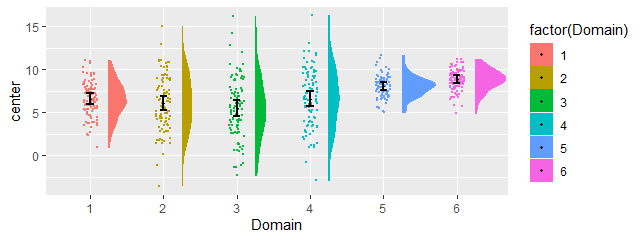Figure 2, version with colors

## Adding graphic directives for fine-tuning the plot

We can customize any superb plot by adding graphic directives one-by-one using the operator +, or we can collect all the directives in a list, and add this list once. As we have two plots with mostly the same directives, we use this second approach.

Typically, a plot is customized by picking a theme. The default theme_bw() is grayish, so we move to theme_classic(). We also customize specific aspects of this theme with theme() directives.

These changes are all collected within the list commonstyle below:

commonstyle <- list(
theme_classic(),                             # It has no background, no bounding box.

# We customize this theme further:
theme(axis.line=element_line(size=0.50),     # We make the axes thicker...
axis.text = element_text(size = 10),     # their text bigger...
axis.title = element_text(size = 12),    # their labels bigger...
plot.title = element_text(size = 10),    # and the title bigger as well.
panel.grid = element_blank(),            # We remove the grid lines
legend.position = "none"                 # ... and we hide the side legend.
),

# Finally, we place tick marks on the units
scale_y_continuous( breaks=1:10 ),

# set the labels to be displayed
scale_x_discrete(name="Domain", labels = mylabels),

# and set colours to both colour and fill layers
scale_discrete_manual(aesthetic =c("fill","colour"), values = mycolors)
)
## Warning: The size argument of element_line() is deprecated as of ggplot2 3.4.0.
## i Please use the linewidth argument instead.

We also changed the vertical scale (tick marks at designated positions) and the horizontal scale with names on the tick marks (sadly, superb replaces them with consecutive numbers…) and colors to fill the clouds (fill) and their borders (colour) as well as the rain drop colors.

Examining this plot with the commonstyle added, we get

finalpltA <- pltA + aes(fill = factor(Domain), colour = factor(Domain)) +
commonstyle +                           # all the above directive are added;
coord_cartesian( ylim = c(1,10) ) +     # the y-axis bounds are given ;
labs(title="A") +                       # the plot is labeled "A"...
ylab("Self-worth relevance")            # and the y-axis label given.
finalpltA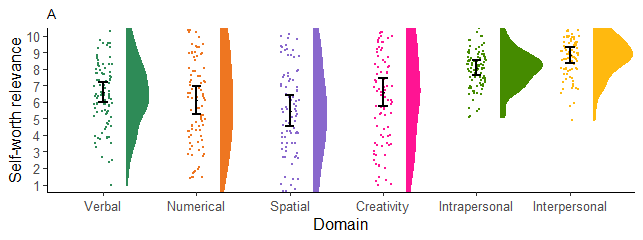Figure 2, final version

## Making the second row of the figure

We do exactly the same for the second plot. We just change the data set to dtaB and in the last graphic directives, using options tailored specifically to this second data set (smaller y-axis range, different label, etc.):

pltB <- superbPlot(dtaB,        # plot for the second data set...
WSFactors = "Domain(6)",    # ...a within-subject design with 6 levels
variables = mylabels,       # ...whose variables are contained in the above list
purpose = "difference", # we want to compare means
decorrelation = "CM"    # and error bars are correlated-adjusted
),
plotStyle="raincloud",
# the following (optional) arguments are adjusting some of the visuals
pointParams    = list(size = 0.75),
jitterParams = list(width =0.1, shape=21,size=0.05,alpha=1), # less dispersed jitter dots,
violinParams = list(trim=TRUE, alpha=1,adjust=3),            # not semi-transparent, smoother
errorbarParams = list(width = 0.1, size=0.5)                 # wider bars, thicker lines.
)
## Warning in (function (mapping = NULL, data = NULL, stat = "identity", position =
## "identity", : Ignoring unknown parameters: size
finalpltB <- pltB + aes(fill = factor(Domain), colour = factor(Domain)) +
commonstyle +                           # the following three lines are the differences:
coord_cartesian( ylim = c(1,5) ) +      # the limits, 1 to 5, are different
labs(title="B") +                       # the plot is differently-labeled
ylab("Judgment certainty")              # and the y-axis label differns.
finalpltB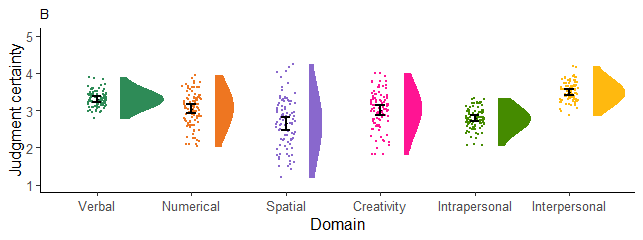Figure 2, bottom row

## Combining and saving both plots

Finally, we assemble the two plots together

finalplt <- grid.arrange(finalpltA, finalpltB, ncol=1)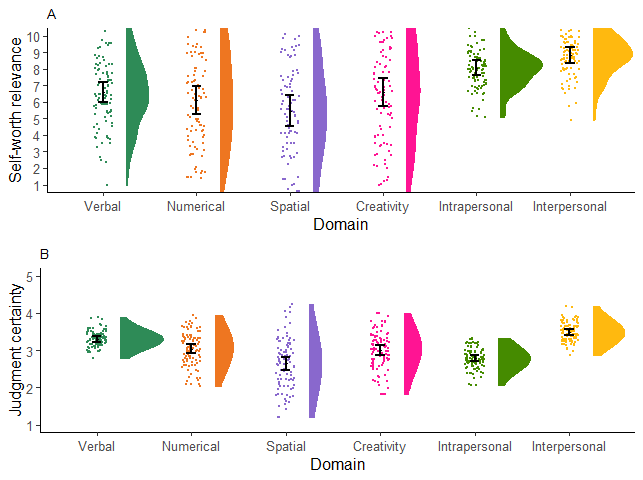Figure 2, final version

It can be saved with high-resolution if desired with

ggsave( "Figure2.png",
plot=finalplt,
device = "png",
dpi = 320,          # pixels per inche
units = "cm",       # or "in" for dimensions in inches
width = 17,         # as found in the article
height = 13
)

That’s it!